Next: Numerical Methods Up: Equations of Motion Previous: The Orbits

## Calculating the Equation of Motion

Now we have all the tools and material ready to deduce the equations of motion for the system of particles in an expanding universe.

Even though the parameterized orbits in equation (3.21) are different from the paths from Hamilton's principle, they behave similarly in the action A from equation (3.1). We can therefore use the same deduction as we did in Section 3.2. The rewritten version of equation (3.5), satisfying the notation of the current case, isThe next step now is to insert the Lagrangian from equation (3.20), which results in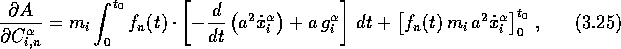where the peculiar gravitational acceleration is given by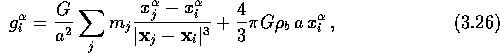measured by a comoving observer at rest relative to distant matter. Note that the second term in equation (3.26) cancel the first in the limit of a homogeneous mass distribution.

As discussed in section 3.2, the last term in equation (3.25) cancel out when the path is fixed at the end-points; i.e. the variation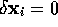at t=0 and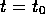. As mentioned, P-89 showed that also mixed boundary conditions can be applied, which he gave asThe first condition is the same as in Hamilton's principle; i.e. the variation vanishes. It is with the second condition that we get a deviation. It indicates that the peculiar velocities vanish as one gets close to the primeval area, which is in correspondence with the gravitational instability picture. By comparing these boundary values to the last term in equation (3.25), one can easily see that the former cancels the latter. Hence, Hamilton's principle, or in this case, the AVP applies.

The resulting derivative of the action isThus, we see that orbits that satisfy the set of equations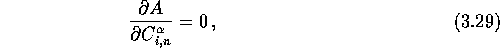satisfy time averages of the usual cosmological equations of motion.

With the assistance of the fundamental theorem of variational calculus (see Section 3.2), we have the equations of motion given by the parameters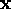,, and t:When dealing with problems in cosmology, it is often convenient to consider the expansion parameter as a measure of time instead the usual SI-unit, seconds. It is even more convenient if one assumes the expansion parameter to be zero at the Big Bang,=0) = 0, and unity at the present,=. The transformation from time to scale factor in the matter dominated epoch of the Universe is given by the Friedmann-equations: In the case considered here, where the Universe is cosmologically flat (k=0), have zero pressure, and a non-negligible cosmological constant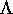, it is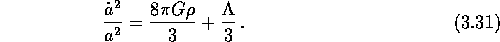The productis constant throughout the history of the Universe; thus, we have. The Friedmann equations also tell us thatwhen k=0.If we include these two expressions, and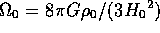, in equation (3.31), we arrive at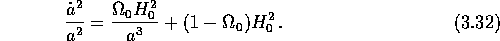After rearranging this equation, we get an expression for the time interval given by an interval in the expansion parameterA similar expression for a curved universe with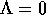can be derived:The only difference between equation (3.33) and (3.34) is the exponent of a in the denominator. Only the former equation will be considered here.

Inserting equation (3.33) into equation (3.30) gives us the equation of motion expressed in the parameters,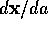, and a:whereis the present radius of a sphere that would contain the total massof particles in the solution if the mass density was homogeneous.is given by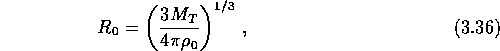where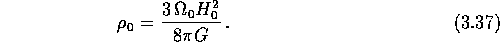I close this section by noting that the boundary conditions in equation (3.27) can be arbitrary and are not restricted to the form needed by the AVP, since they were only used to remove the integrated part in equation (3.25). The application of equation (3.28) is therefore more general than that of the action principle itself. It is then possible to to use redshifts instead of current positions when calculating the orbits Gia,P-94.Next: Numerical Methods Up: Equations of Motion Previous: The Orbits

Trond Hjorteland
Mon Jul 5 02:59:28 MET DST 1999